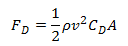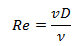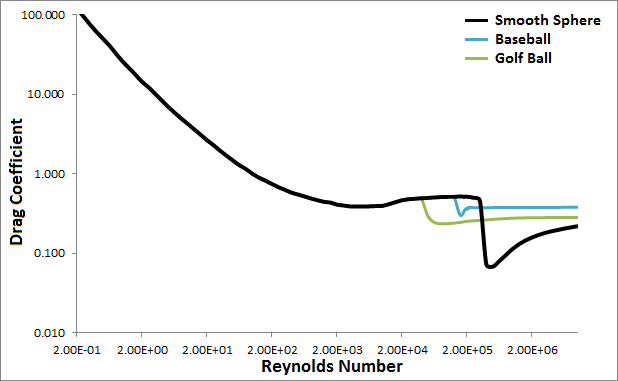# Projectile Drag

The force of drag (or air resistance) on an object is in the opposite direction of the velocity of that object relative to the air, and can be expressed by,where

F`D` = force of drag

ρ = density of air

v = velocity of the object relative to the air

A = cross-sectional area of the object

C`D` = drag coefficient

In the original VirtualTrebuchet simulation the drag coefficient, C`D`, was assumed to be a constant 0.47. This is a reasonable assumption over a certain velocity range.

In VirtualTrebuchet 2.0, the drag coefficient, C`D`, is not assumed to be a constant. Instead the drag coefficient is continually recalculated during the simulation. It is derived by first finding the Reynolds number of the object at a particular time. The Reynolds number, Re, is non-dimensional number defined as,where

v = velocity of the object relative to the air

D = diameter of the object

ν = the kinematic viscosity of the air

People have found the relationships between the Reynolds number and the drag coefficient for different shapes empirically. For the simulation, I used the charts in Figures 9.21 and 9.25 of the textbook, Fundamentals of Fluid Mechanics Fifth Edition to get the relationship between the Reynolds number and the drag coefficient for a smooth sphere. The following chart shows the approximate shape of this relationship.All the projectiles in the simulation are assumed to be smooth spheres except for the baseball and golf ball options. The simulation uses special curves for the baseball and golf ball as shown in the chart above. The data the simulation uses for the baseball and golf ball came from a paper by Rabindm D. Mehta and Jani Macari Pallis called Sports Ball Aerodynamics: Effects of Velocity, Spin and Surface Roughness (PDF).

 Units English (feet)English (inches)Metric Length of Short Arm ft Length of Long Arm ft Length of Sling ft Length of Weight ft Height of Pivot ft Uniform arm Mass of Arm lb Inertia of Arm lb·ft² Pivot to Arm CG ft Mass of Weight lb Inertia of Weight lb·ft² Projectile BaseballGolf BallPumpkinCowCustom Mass of Projectile lb Projectile Diameter ft Wind Speed ft/s Release Angle ° Play speed: 1x Link to save this trebuchet

## VirtualTrebuchet 2.0

VirtualTrebuchet is a web based trebuchet simulator that will allow you to quickly evaluate different trebuchet configurations.

1. To begin, enter the parameters of your trebuchet in the input boxes.
2. Next, press the "Simulate" button located under the inputs.
3. Finally, watch your trebuchet go.